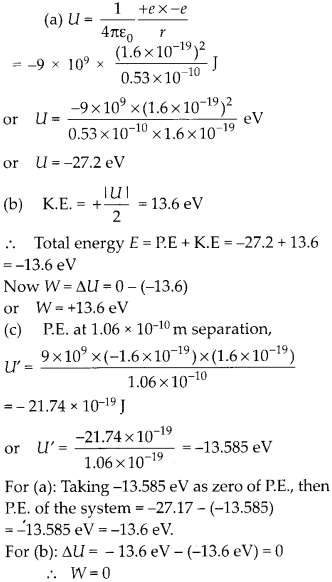Enlightened

# Question 18: NCERT Solutions for 12th Class Physics: Chapter 2-Electrostatic Potential and Capacitance

• 0

Question 18: NCERT Solutions for 12th Class Physics: Chapter 2-Electrostatic Potential and Capacitance

In a hydrogen atom, the electron and proton are bound at a distance of about 0.53 A:
(a) Estimate the potential energy of the system in eV, taking the zero of the potential energy at infinite separation of the electron from proton.
(b) What is the minimum work required to free the electron, given that its kinetic energy in the orbit is half the magnitude of potential energy obtained in (a)?
(c) What are the answers to (a) and (b) above if the zero of potential energy is taken at 1.06 A separation?

Share